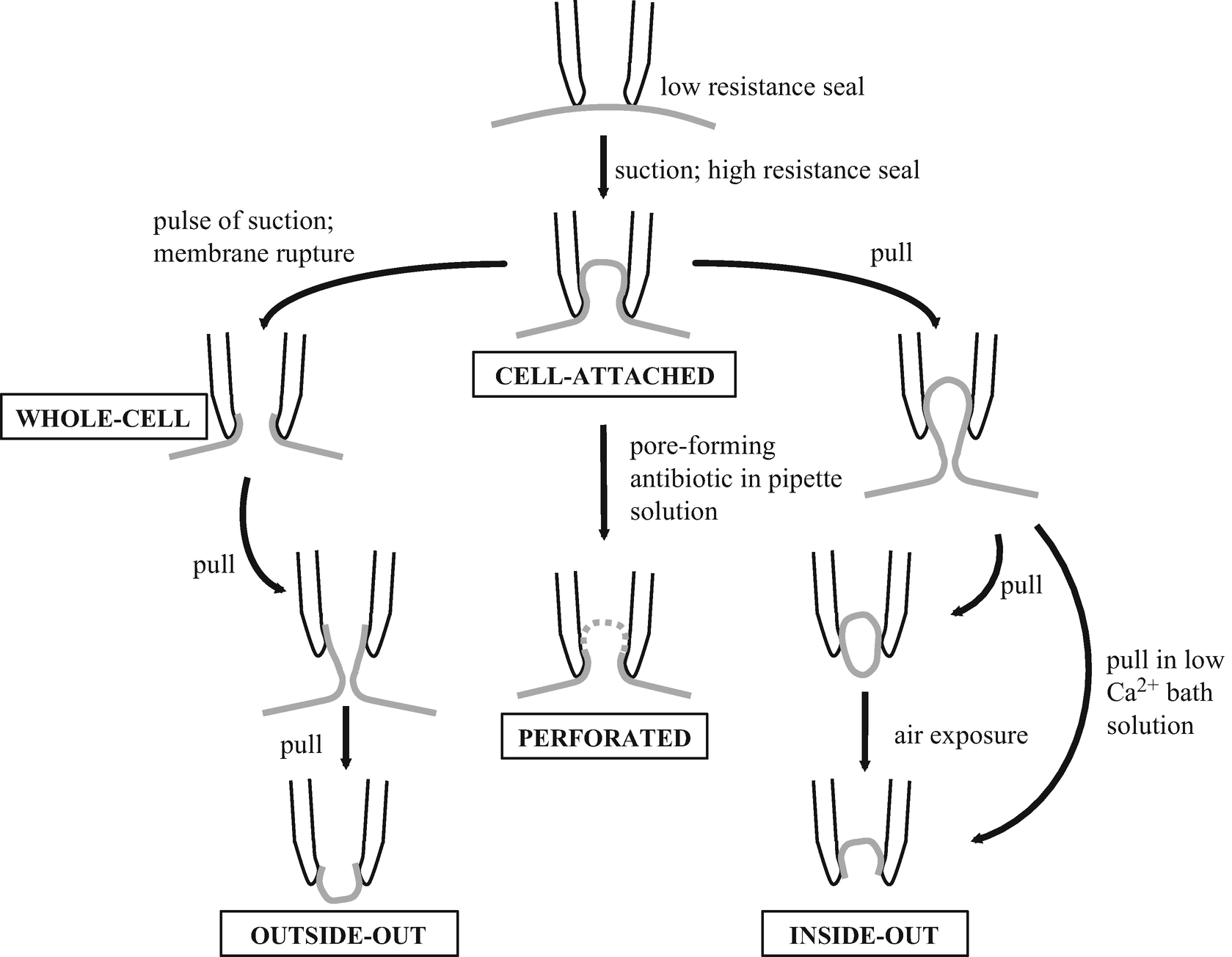## 简介

### 细胞膜及其电特性

$$\Delta E=-\frac{RT}{ZnF}\ln\left({a_{n}}^{A}/{a_{n}}^{B}\right) \tag{能斯特方程}$$

$$I=\frac VR \tag{欧姆定律}$$

## 膜片钳记录的方法### 膜片钳记录中的电力学原理及注意事项